Courses

# Previous year Questions (2016 - 20) Notes | EduRev

## Class 11 : Previous year Questions (2016 - 20) Notes | EduRev

The document Previous year Questions (2016 - 20) Notes | EduRev is a part of Class 11 category.
All you need of Class 11 at this link: Class 11

Q.1. The ammonia (NH3) released on quantitative reaction of 0.6 g urea (NH2CONH2) with sodium hydroxide (NaOH) can be neutralized by 
(1) 200 mL of 0.4 N HCl
(2) 200 mL of 0.2 N HCl
(3) 100 mL of 0.2 N HCl
(4) 100 mL of 0.1 N HCl
Ans. (3)
Solution.
From the stoichiometry of the reaction,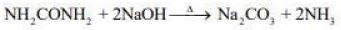1 mol of urea liberates 2 moles of ammonia
Moles of urea = 0.6 / 60 = 0.01 mol
Thus, moles of ammonia formed = 2 × 0.01 = 0.02 mol
Since, 1 mol HCl is required to neutralized 1 mol of ammonia.
So, 0.02 moles of HCl is required to neutralized 0.02 moles of ammonia.
From the given concentration of HCl, 100 mL of 0.2 N is equivalent to 0.02 moles of ammonia.
⇒ Moles of HCl = 0.1 × 0.2 = 0.02 moles

Q.2. Ferrous sulphate heptahydrate is used to fortify foods with iron. The amount (in grams) of the salt required to achieve 10 ppm of iron in 100 kg of wheat is _________. (2020)
Atomic weight: Fe = 55.85; S = 32.00; O = 16.00
Ans.
(4.95)
Solution. The mass of the ferrous sulphate heptahydrate (FeSO4. 7H2O) = 277.55 g mol-1
⇒ 277.55 g of (FeSO4 . 7H2O) 55.85 g of Fe is present.
⇒ 1 g of Fe is present in = 277.55 / 55.85 g of FeSO4 . 7H2O
⇒1 g of Fe is present in = 4.97 g of FeSO4.7H2O

Q.3. The molarity of HNO3 in a sample which has density 1.4 g mL-1 and mass percentage of 64% is _____. (Molecular weight of HNO3 = 63). (2020)
Ans.
(14)
Solution. Given, ρ =1.4 g mL-1 and mass percentage = 63%
We know,
Molarity (M) =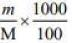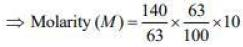m =  ρ x V
= 1.4 x 1000
= 140 g
⇒ Molarity (M) = 14 mol L-1

Q.4. 10.30 mg of O2 is dissolved into a liter of sea water of density 1.03 g mL-1. The concentration of O2 in ppm is_____. (2020)
Ans.
(10.0)
Solution. The concentration of dissolved oxygen in water is
DO in water (ppm) = Amount of oxygen / Amount of water
=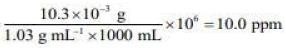Q.5. Amongst the following statements, that which was not proposed by Dalton was (2020)
(1) chemical reactions involve reorganization of atoms. These are neither created nor destroyed in a chemical reaction.
(2) all the atoms of a given element have identical properties including identical mass. Atoms of different elements differ in mass.
(3) when gases combine or reproduced in a chemical reaction, they do so in a simple ratio by volume provided all gases are at the same T and P.
(4) matter consists of indivisible atoms.

Ans. (3) The statement (3) is incorrect and the correct statement is :
When atoms of different elements combine to form compounds, new and more complex particles form.
However, in a given compound the constituent atoms are always present in the same fixed numerical ratio.

Q.6. The strength of an aqueous NaOH solution is most accurately determined by titrating (Note: Consider that an appropriate indicator is used) (2020)
(1) Aq. NaOH in a pipette and aqueous oxalic acid in a burette.
(2) Aq. NaOH in a burette and aqueous oxalic acid in a conical flask.
(3) Aq. NaOH in a burette and concentrated H2SO4 in a conical flask.
(4) Aq. NaOH in a volumetric flask and concentrated H2SO4 in conical flask.
Ans.
(2)
Solution. The strength of aq. NaOH solution is most accurately determined by titration with standard oxalic acid. Keeping aq. NaOH in burette and oxalic acid in conical flask.

Q.7. NaClO3 is used, even in spacecrafts, to produce O2. The daily consumption of pure O2 by a person is 492 L at 1 atm, 300 K. How much amount of NaClO3, in grams, is required to produce O2 for the daily consumption of a person at 1 atm, 300 K? (2020)
NaClO3(s) + Fe(s) → O2(g) + NaCl(s) + FeO(s)

R = 0.082 L atm mol−1 K−1
Ans.
(2130)
Solution. The given reaction is
NaClO3(s) + Fe(s) → FeO(s) + NaCl(s) + O2(g)
From the above reaction,
1 mol of oxygen is produced by 1 mol of NaClO3,
16 g of oxygen is produced by 106.5 g of NaClO3,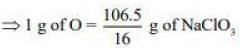Amount of O2 required by a person in a day in grams can be calculated by ideal gas equation,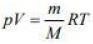⇒ m = pVM / RT
Substituting the values in Eq. (1), we get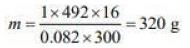Thus, 320 g of oxygen is produced by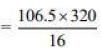2130 g of NaClO3.

Q.8. The mass percentage of nitrogen is histamine is _________. (2020)
Ans.
(37.80)
Solution. The structural formula of histamine is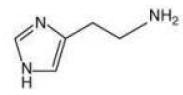The molar mass of histamine (C5H9N3) = 111.15 g mol–1
Thus, the mass percentage of nitrogen in histamine

Mass percentage of N = Mass of nitrogen /  Total mass of histamine
⇒ Mass percentage of N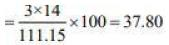Q.9. The hardness of a water sample containing 10−3 M MgSO4 expressed as CaCO3 equivalents (in ppm) is____. (2020)
(Molar mass of MgSO4 is 120.37 g mol–1)
Ans.
(100)
Solution. We know,
10–3 M of MgSO4 = 0.001 moles of MgSO4 present in 1 L water.
Since, number of moles of MgSO4 and CaCO3 are equal, that is, nMgSO4 = nCaCO3 .
Thus, hardness of water sample in expressed as CaCOequivalents in ppm is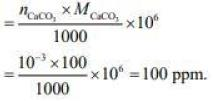Q.10. 5 g of zinc is treated separately with an excess of
(I) dilute hydrochloric acid and
(II) aqueous sodium hydroxide
The ratio of the volumes of Hevolved in these two reactions is (2020)

(1) 1 : 2
(2) 1 : 1
(3) 1 : 4
(4) 2 : 1
Ans.
(2)
Solution. The moles of zinc nza = m / M = 5 / 65.38 = 0.076 mol
The reaction of zinc with HCI and NaOH is
Zn(s) + 2HCl(aq) → ZnCl2(aq) + H2(g)
Zn(s) + 2NaOH(aq) + 2H2O(l) → Na2Zn(OH)4(aq) + H2(g)
1 mol of Zn react with HCI and NaOH respectively, 1 mol of hydrogen gas is liberated from each reaction. Thus, the ratio of hydrogen gas evolved when 5 g of Zn reacts with dil. HCI and aq. NaOH solutions is 1 : 1.

Q.11. The redox reaction among the following is (2020)
(1) Formation of ozone from atmospheric oxygen in the presence of sunlight
(2) Reaction of [Co(H2O)6]Cl3 with AgNO3
(3) Reaction of H2SO4 with NaOH
(4) Combination of dinitrogen with dioxygen at 2000 K
Ans.
(4)
Solution. The reaction in which both oxidation and reduction takes place are called redox reaction. Amongst the given, option (4) is a example of redox reaction.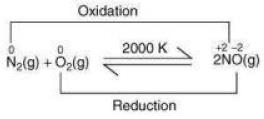Q.12. A solution of sodium sulphate contains 92 g of Na+ ions per kilogram of water. The molality of Na+ ions in that solution in mol kg-1 is: (2019)
(1) 12
(2) 4
(3) 8
(4) 16
Ans.
(2)
Solution. Number of moles in 92 g of Na= 92 / 23 = 4 moles
Molality (m) = Number of moles / Mass of solvent (in Kg)
∴ m = 4 / 1 = 4 mol kg-1

Q.13. For the following reaction the mass of water produced from 445 g of C57H110O6 is: (2019)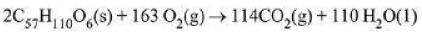(1) 490 g
(2) 445 g
(3) 495 g
(4) 890 g
Ans.
(3)
Solution. For the given reaction:n = 445 / 890 = 0.5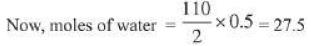∴ Mass of water = 27.5 x 18 = 495 g

Q.14. A mixture of 100 milli mol of Ca(OH)2 and 2 g of sodium sulphate was dissolved in water and the volume was made up to 100 mL. The mass of calcium sulphate formed and the concentration of OH- in resulting solution, respectively, are: (Molar mass of Ca(OH)2, Na2S04 and CaS04 are 74, 143 and 136 g mol-1, respectively; Ksp of Ca(OH)2 is 5.5 x 10-6) (2019)
(1) 1.9 g, 0.28 mol L-1
(2) 13.6 g, 0.28 mol L-1
(3) 1.9 g, 0.14 mol L-1
(4) 13.6 g, 0.14 mol L-1
Ans.
(1)
Solution.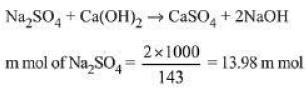m mol of  CaSO2 formed = 13.98 m mol
Mass of CaSO2 formed
= 13.98 x 10-3 x 136 = 1.90 g
m mol of NaOH = 28 m mol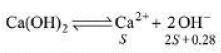Value of 'S' will be negligible so
[OH-] = 0.028 / 0.1 = 0.28 mol L-1

Q.15. A 10 mg effervescent tablet containing sodium bicarbonate and oxalic acid releases 0.25 mL of CO2 at T = 298.15K and P = 1 bar. If molar volume of CO2 is 25.0 L under such condition, what is the percentage of sodium bicarbonate in each tablet? (2019)
[Molar mass of NaHCO3 = 84 g mol-1]
(1) 0.84
(2) 33.6
(3) 16.8
(4) 8.4
Ans.
(4)
Solution.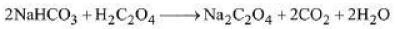Moles of CO2 evolved =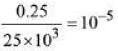∴ Moles of NaHCO3 = 10-5
∴ Moles of NaHCO3 = 84 x 10-5 g
= 0.84 x 10-3 g
% by weight =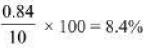Q.16. 25 mL of the given HCI solution requires 30 mL of 0.1 M sodium carbonate solution. What is the volume of this HCI solution required to titrate 30 mL of 0.2 M aqueous NaOH solution? (2019)
(1) 25 mL
(2) 75 mL
(3) 50 mL
(4) 12.5 mL
Ans.
(1)
Solution. 25 mL of HCI solution requires 30 mL of 0.1 M Na2CO3 solution.
∴ N1V1 = N2V2
∴ 25 x N1 = 30 x 0.2 (0.1 M Na2CO3 = 0.2N Na2 CO3)
N1 = 6 / 25 = 0.24N
Now, HCI solution is titrated with NaOH solution.
M1V1 = M2V2 ; 0.24 N HCl = 0.24 M HCl
∴ V x 0.24 x 1 = 30 x 0.2 x 1 ⇒ V = 25 mL

Q.17. 50mL of 0.5 M oxalic acid is needed to neutralize 25 mL of sodium hydroxide solution. The amount of NaOH in 50 mL of the given sodium hydroxide solution is: (2019)
(1) 40 g
(2) 10 g
(3) 20 g
(4) 80 g
Ans.
Bonus (No option is correct)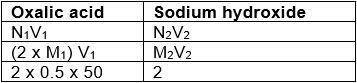Molarity = No. of moles / Vol.(L)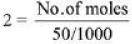No. of moles = 1 / 10 = 0.1
No. of moles = W / Molar mass
W = 0.1 x 40 = 4 g

Q.18. For a reaction, N2(g) + 3H2(g) → 2 NH3(g); identify dihydrogen (H2) as a limiting reagent in the following reaction mixtures. (2019)
(1) 56 g of N2 + 10 g of H2
(2) 35 g of N2 + 8 g of H2
(3) 28 g of N2 + 6 g of H2
(4) 14 g of N2 + 4 g of H
Ans.
(1)
Solution. According to the stoichiometry of balanced equation 28 g N2, react with 6 g H2,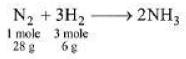∴ For 56 g of N2, 12 g of H2 is required.

Q.19. At 300 K and 1 atmospheric pressure, 10 mL of a hydrocarbon required 55 mL of O2 for complete combustion, and 40 mL of CO2 is formed. The formula of the hydrocarbon is: (2019)
(1) C4H10
(2) C4H6
(3) C4H7C1
(4) C4H8
Ans. (2)
Solution. Let the hydrocarbon be CxHy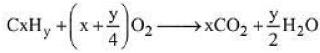Before Combustion : 10 mL, 55 mL, 0
After combustion : 0, 55 - 10(x + y / 4), 10x
Volume of CO2, 10x = 40; x = 4
55 - 10(x + y / 4) = 0; y = 6
∴ Hydrocarbon is C4H6

Q.20. The minimum amount of O2(g) consumed per gram of reactant is for the reaction:
(Given atomic mass: Fe = 56, O= 16, Mg = 24, P = 31, C = 12, H = 1) (2019)

(1) 4 Fe(s) + 3O2(g) → 2 Fe2O3 (s)
(2) P4(s) + 5O2(g) → P4O10(s)
(3) C3H8(g) + 5O2(g) → 3CO2(g) + 4H2O(I)
(4) 2Mg(s) + O2(g) → 2MgO(s)
Ans.
(1)
Solution.
(1) 4 Fe + 3O2 → 2 Fe2O3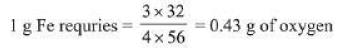(2) P4 + 5O2 → P4O10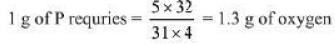(3) C3H8 + 5O2 → 3CO2 + 4H2O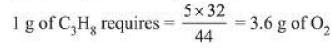(4) 2Mg + O2 → 2MgO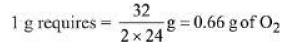Q.21. 5 moles of AB2 weigh 125 x 10-3 kg and 10 moles of A2B2 weigh 300 x 10-3 kg. The molar mass of A (MA) and molar mass of B (MB) in kg mol-1 are: (2019)
(1) MA= 10 x 10-3 and MB = 5 x 10-3
(2) MA= 50 x 10-3 and MB= 25 x 10-3
(3) MA = 25 x 10-3 and MB= 50 x 10-3
(4) MA = 5 x 10-3 and MB = 10 x 10-3
Ans.
(4)
Solution. 5 mol AB, weighs 125 g
∴ AB2 = 25 g / mol
10 mol A2B2 weighs 300 g
∴ A2B2 = 30 g / mol
∴ Molar mass of A(MA) = 5 g or 5 x 10-3 kg
Molar mass of A(MA) = 10 g or 10 x 10-3 kg

Q.22. Consider the following reduction processes:
Zn2+ + 2e- → Zn(s); E° = - 0.76 V
Ca2+ + 2e- → Ca(s); E° = - 2.87 V
Mg2+ + 2e- → Mg(s); E° = - 2.36 V
Ni2+ + 2e- → Ni(s); E° = - 0.25 V
The reducing power of the metals increases in the order: (2019)
(1) Ca < Zn < Mg < Ni
(2) Ni < Zn < Mg < Ca
(3) Zn < Mg < Ni < Ca
(4) Ca < Mg < Zn < Ni

Ans. (2)
Solution. Higher the oxidation potential, higher will be the reducing power. So, the order of reducing behaviour is: Ca > Mg > Zn > Ni.

Q.23. In the reaction of oxalate with permanganate in acidic medium, the number of electrons involved in producing one molecule of CO2 is: (2019)
(1) 1
(2) 10
(3) 2
(4) 5
Ans. (1)
Solution.
Reaction involved :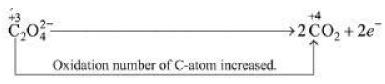∴ The number of electrons involved in producing one mole of CO2 is 1.

Q.24. In order to oxidise a mixture of one mole of each of FeC2O4, Fe2(C2O4)3, FeSO4 and Fe2(SO4)3 in acidic medium, the number of moles of KMnO4 required is: (2019)
(1) 2
(2) 1
(3) 3
(4) 1.5
Ans.
(1)
Solution.
MnO-4 + 5e- → Mn2+
(i) FeC2O→ Fe3+ + 2CO2 + 3e-
1 mole of FeC2Oreacts with 3 / 5 mole of acidified KMnO4
(ii) Fe2(C2O4)3 → Fe3+ + 2CO2 + 6e-
1 mole of Fe2(C2O4)3 reacts with 6 / 5 mole of acidified KMnO4
(iii) FeSO4 →  Fe3+ + e-
1 mole of FeSO4 reacts with 1 / 5 mole of acidified KMnO4
(iv) Fe2(SO4)3 does not oxidise
∴ Total moles required = 3 / 6 + 6 / 5 + 1 / 5 = 2

Q.25. An example of a disproportionation reaction is: (2019)
(1) 2MnO4+ 10I- + 16H+ → 2Mn2+ + 5I2 + 8H2O
(2) 2NaBr + Cl2 → 2NaCl + Br2
(3) 2KMnO4 → K2MnO+ MnO2 + O2
(4) 2CuBr → CuBr2 + Cu
Ans.
(4)
Solution.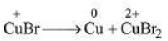It is an example of disproportionation reaction, as Cu undergoes both oxidation and reduction.

Q.26. The oxidation states of Cr in [Cr(H2O)6] Cl3 , [Cr(C6H6)2] and K2[Cr(CN)2 (O)2 (O2) NH3 ] respectively are: (2018)
(1) +3, +4, and +6
(2) +3, +2, and +4
(3) +3, 0, and +6
(4) +3, 0, and +4
Ans.
(3)
Solution. The Oxidation state of Cr in

[Cr(H2O)6
Cl3 x + 6(0) - 3 = 0
x = +3

[Cr(C6H6)2]

x + 2(0) = 0
x = 0
K2[Cr(CN)2 (O)2 (O2) NH3
+2 + x + 2 (-1) + 2 (-2) +1 (-2) + 0
= 6

∴ x = +6

Q.27. A sample of NaCIO3 is converted by heat to NaCl with a loss of 0.16 g of oxygen. The residue is dissolved in water and precipitated as AgCl. The mass of AgCI (in g) obtained will be: (Given: Molar mass of AgCl= 143.5 g mol-1) (2018
(1) 0.35
(2) 0.48
(3) 0.54
(4) 0.41
Ans.
(2)
Solution.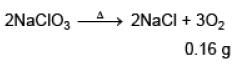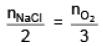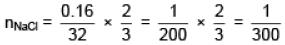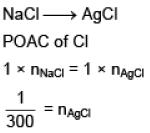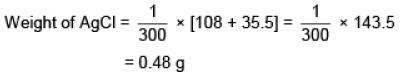Q.28. An unknown chlorohydrocarbon has 3.55 % of chlorine. If each molecule of the hydrocarbon has one chlorine atom only ; chlorine atoms present in 1 g of chlorohydrocarbon are: (2018)
(Atomic wt. of Cl = 35.5 u ; Avogadro constant = 6.023 × 1023 mol–1)
(1) 6.023 × 109
(2) 6.023 × 1023
(3) 6.023 × 1021
(4) 6.023 × 1020
Ans.
(4)
Solution. CxHyCl
% Cl = 3.55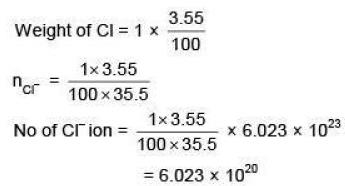Q.29. The most abundant elements by mass in the body of a healthy human adult are:
Oxygen (61.4%); Carbon (22.9%); Hydrogen (10.0%) and Nitrogen (2.6%).

The weight which a 75 kg person would gain if all 1H atoms are replaced by 2H atoms is (2017)
(1) 15 kg
(2) 37.5 kg
(3) 7.5 kg
(4) 10 kg
Ans. (3)
Solution. Mass of hydrogen = 10 / 100 x 75 = 7.5 kg
Replacing 1H by 2H would replace 7.5 kg with 15 kg
∴ Net gain = 7.5 kg

Q.30. 1 gram of a carbonate (M2CO3) on treatment with excess HCl produces 0.01186 mole of CO2. The molar mass of M2CO3 in g mol-1 is (2017)
(1) 1186
(2) 84.3
(3) 118.6
(4) 11.86
Ans. (2)
Solution. M2CO3 + 2HCl → 2MCl + H2O + CO2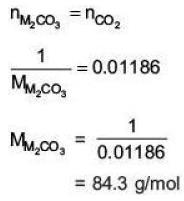Q.31. Excess of NaOH(aq) was added to 100 mL of FeCI3(aq) resulting into 2.14 g of Fe(OH)3. The molarity of FeCI3(aq) is:
(Given molar mass of Fe = 56 g mol-1 and molar mass of CI = 35.5 g mol-1) (2017)

(1) 0.3 M
(2) 0.2 M
(3) 0.6 M
(4) 1.8 M
Ans. 2
Solution.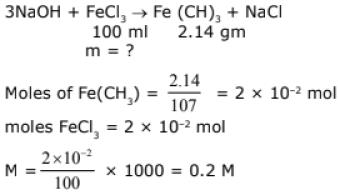Q.32. What quantity (in mL) of a 45% acid solution of a mono-protic strong acid must be mixed with a 20% solution of the same acid to produce 800 mL of a 29.875% acid solution? (2017)
(1) 316
(2) 320
(3) 325
(4) 330
Ans.
(1)
Solution.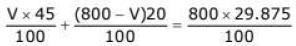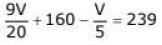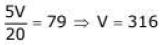Q.33. At 300 K and 1 atm, 15 mL of a gaseous hydrocarbon requires 375 mL air containing 20% O2 by volume for complete combustion. After combustion the gases occupy 330 mL. Assuming that the water formed is in liquid form and the volumes were measured at the same temperature and pressure, the formula of the hydrocarbon is: (2016)
(1) C3H6
(2) C3H8
(3) C4H8
(4) C4H10
Ans.
(2)
Solution.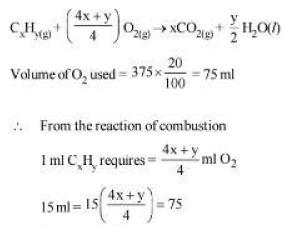So, 4x + y = 20
x = 3
y = 8
C3H8

Q.34. 5L of an alkane requires 25 L of oxygen for its complete combustion.
If all volumes are measured at constant temperature and pressure, the alkane is: (2016)
(1) Ethane
(2) Propane
(3) Butane
(4) Isobutane
Ans.
(2)
Solution. Combustion of Hydrocarbon :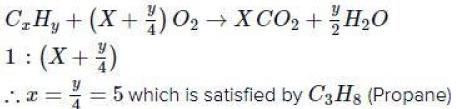Q.35. The amount of arsenic pentasulphide that can be obtained when 35.5 g arsenic acid is treated with excess H2S in the presence of conc. HCl (assuming 100% conversion) (2016)
(1) 0.25 mol
(2) 0.125 mol
(3) 0.333 mol
(4) 0.50 mol
Ans.
(2)
Solution.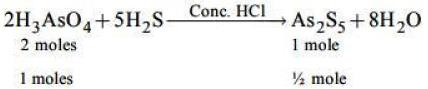∴ number of moles of H3AsO4
= 35.5 / 142 =0.25
∴ number of moles of As2S5
= 0.25 / 2 = 0.125 mole.

Q.36. The volume of 0.1 N dibasic acid sufficient to neutralize 1 g of a base that furnishes 0.04 mole of OH in aqueous solution is : (2016)
(1) 400 mL
(2) 600 mL
(3) 200 mL
(4) 80 mL
Ans.
(3)
Solution. As we know that in a basic acid there is one ionizable hydrogen. While in the dibasic acid there are two ionizable hydrogen. In the given data there are 0.04 moles of OH- ion in aqueous solution. The volume of the solution is found below :
V x 0.1 x 2 = 0.04
V = 200 ml

Offer running on EduRev: Apply code STAYHOME200 to get INR 200 off on our premium plan EduRev Infinity!

,

,

,

,

,

,

,

,

,

,

,

,

,

,

,

,

,

,

,

,

,

;